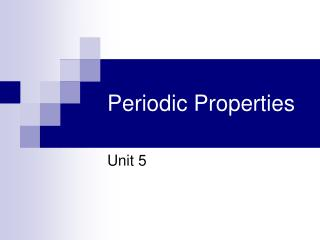Download PresentationPeriodic Properties

# Periodic Properties

Download Presentation## Periodic Properties

- - - - - - - - - - - - - - - - - - - - - - - - - - - E N D - - - - - - - - - - - - - - - - - - - - - - - - - - -
##### Presentation Transcript

1. Periodic Properties Unit 5

2. Pre-Lab Write-Up • The following are due before you can do the lab: • Title • Purpose (be specific) • Materials (optional) • Procedure (be specific) • Data Table(s)

3. Post-Lab Write-Up • The following will be completed once you have completed the lab: • Data Analysis/Calculations • Graph • Conclusion

4. Data Analysis/Calculations • Show calculation for the density of each element, include proper unit, and watch for sig. figs. • Density = mass (g) / ∆volume (mL) • Calculate the percent error of the density of Ge and C. (this will be done after making the graph). Exp - Act Act % Error = x 100 Act. Density of C = 1.4 g/mL Act. Density of Ge = 5.3 g/mL

5. Graph • Construct a graph of density vs. atomic number (plot density on the y-axis and atomic number on the x-axis; both axes MUST start at zero.) • Draw a best fit line through your data • Use the graph to interpolate the density of Ge and extrapolate the density of C • Include appropriate markings on the graph for the unknown densities • Follow all graphing techniques discussed in class

6. Conclusion • In your conclusion you should examine the results of your lab. • What values did you determine for the densities of Ge and C to be? • How were you able to determine the densities of these two elements? (EXPLAIN) • Were the results close to what they were supposed to be (examine your percent error)? • Why did you have error in your lab; what could you/would you do differently next time?